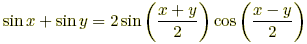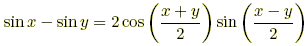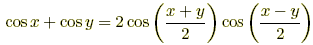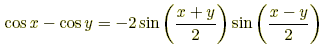trig identities

Trig Identities

Identities involving trig functions are listed below.

Pythagorean Identities

sin2 θ + cos2 θ = 1

tan2 θ + 1 = sec2 θ

cot2 θ + 1 = csc2 θ

 Reciprocal Identities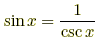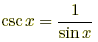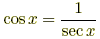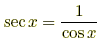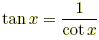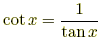Ratio Identities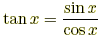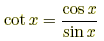Odd/Even Identities sin (–x) = –sin xcos (–x) = cos xtan (–x) = –tan x csc (–x) = –csc xsec (–x) = sec xcot (–x) = –cot x

 Cofunction Identities, radians Cofunction Identities, degrees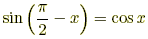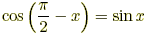sin (90° – x) = cos x cos (90° – x) = sin x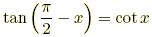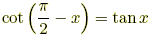tan (90° – x) = cot x cot (90° – x) = tan x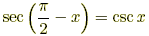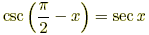sec (90° – x) = csc x csc (90° – x) = sec x

 Periodicity Identities, radians Periodicity Identities, degrees sin (x + 2π) = sin x csc (x + 2π) = csc x sin (x + 360°) = sin x csc (x + 360°) = csc x cos (x + 2π) = cos x sec (x + 2π) = sec x cos (x + 360°) = cos x sec (x + 360°) = sec x tan (x + π) = tan x cot (x + π) = cot x tan (x + 180°) = tan x cot (x + 180°) = cot x

 Sum/Difference Identities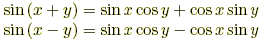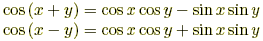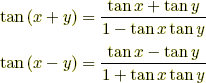Double Angle Identities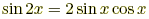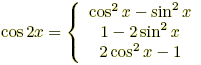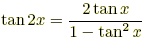Half Angle Identities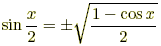or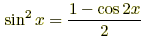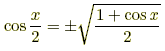or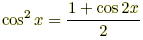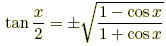or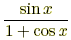or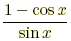Product to Sum Identities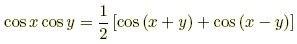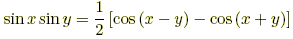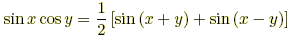Sum to Product Identities